# Team:SCAU-China/Model/CultrueCondition

## Method for optimizing Microbial cell culture

Abstract

To improve the production of bacterial cellulose, it was necessary to increase our cultured cell biomass. In vivo, we have integrated literature data to construct an interactome to help us design a new scheme to realize metabolic engineering. In vitro, based on measured data of Synechocystis growth, we constructed a two layers model to characterize the main parameters influencing the behavior of our system. And find the most important growth factors and find the best culture conditions for Synechocystis.

## Two Layers Model For Microbial Cell Growth Characterized.

We constructed a two layer model to help us characterize microbial cell growth.The basic idea can be decomposed into 'training process' and 'predict process'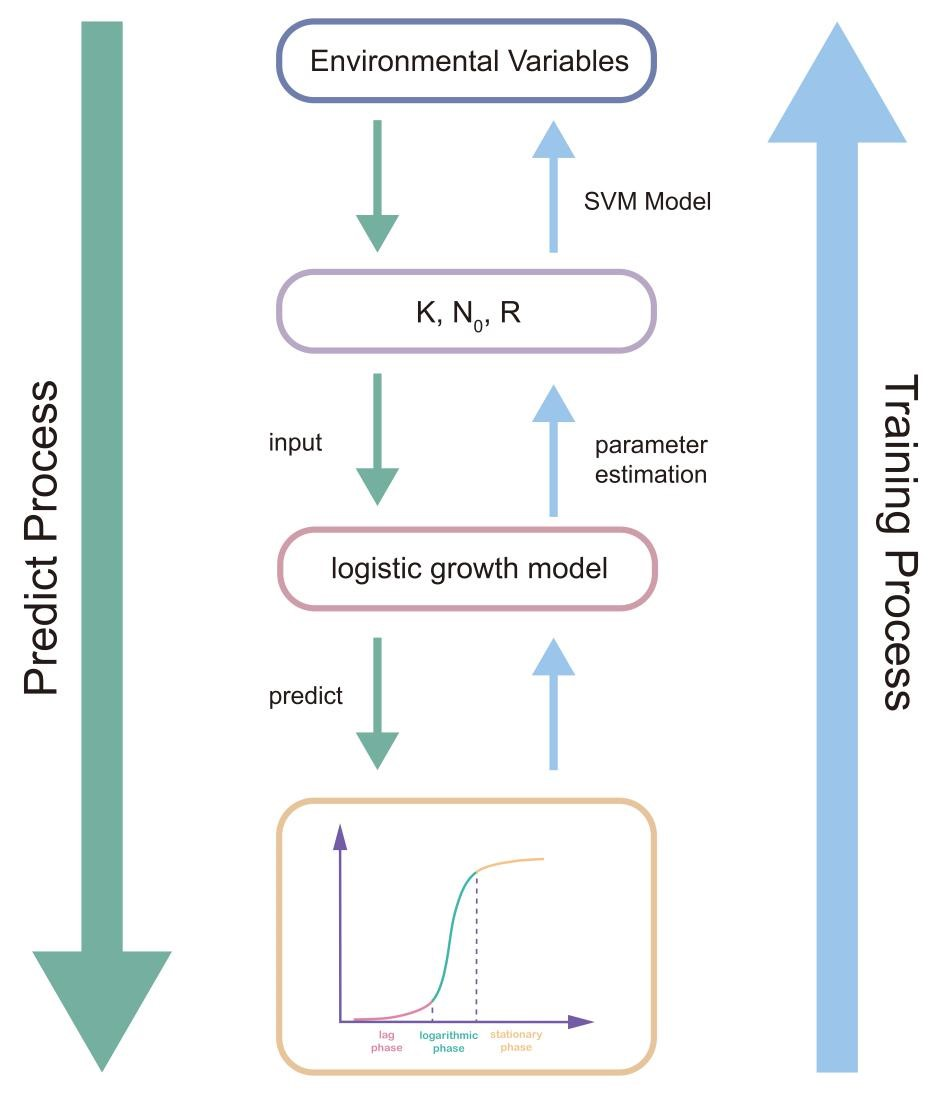## Figure1. The flowchart of our cell growth characterzed model

As shown in Figure1, in the training process, we measured the microbial cell growth data and relevent optimal factors data. After that, we input the microbial cell growth data to the logistic growth model to fit the basic parameters of growth curve. And then, we performed regression model to estimate the effect of factors to the parameters. We used AIC to help us selected model, and we found SVM with linear kernel perform better than optimized multiple regression model when use environment variables(factors) to predict parameters. Predicting process was inverse direction of training process, We input the new culture data and predicted the growth curve of our microbial cell. Compared with real data, we found our model could realize culture conditions optimization.

## Parameter estimation of K,N0,R(The second layer training)

An logistics growth model is used, as shown below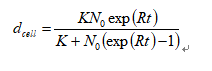We need to fit the parameters K, N0 , R, and we used a useful R package called 'deSolve' to help us to obtain these parameters from measured data. For each sample, we fitted data to derive kinetic parameters and filtered those samples which coefficient of determination(R2) is lower than 0.7 and corresponding variables do not present significant(pvalue> 0.05). Finally, we saved around 12 samples to help us to estimate the first layer parameters and we presented our fit result as shown in below.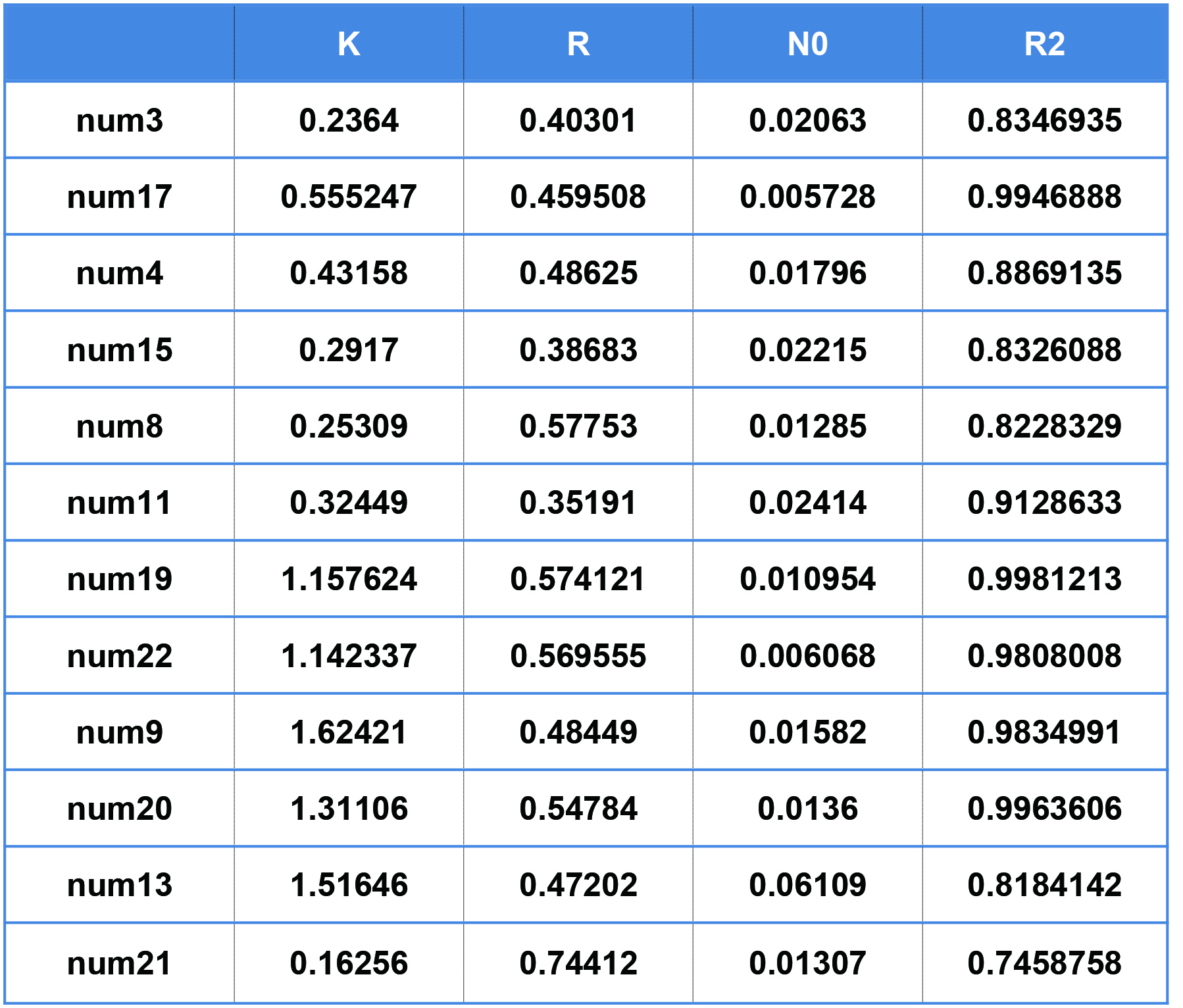## Parameters estimation of culture conditions effects(the first layer training)

After the second layer estimated the parameters and propagated the K, N0, R information to the first layer, we need to train a model to find the relationship between culture condition variable with kinetic parameters. First, we used the multiple regression model to find the culture conditions which have significant influence on our kinetic parameters K,N0,R.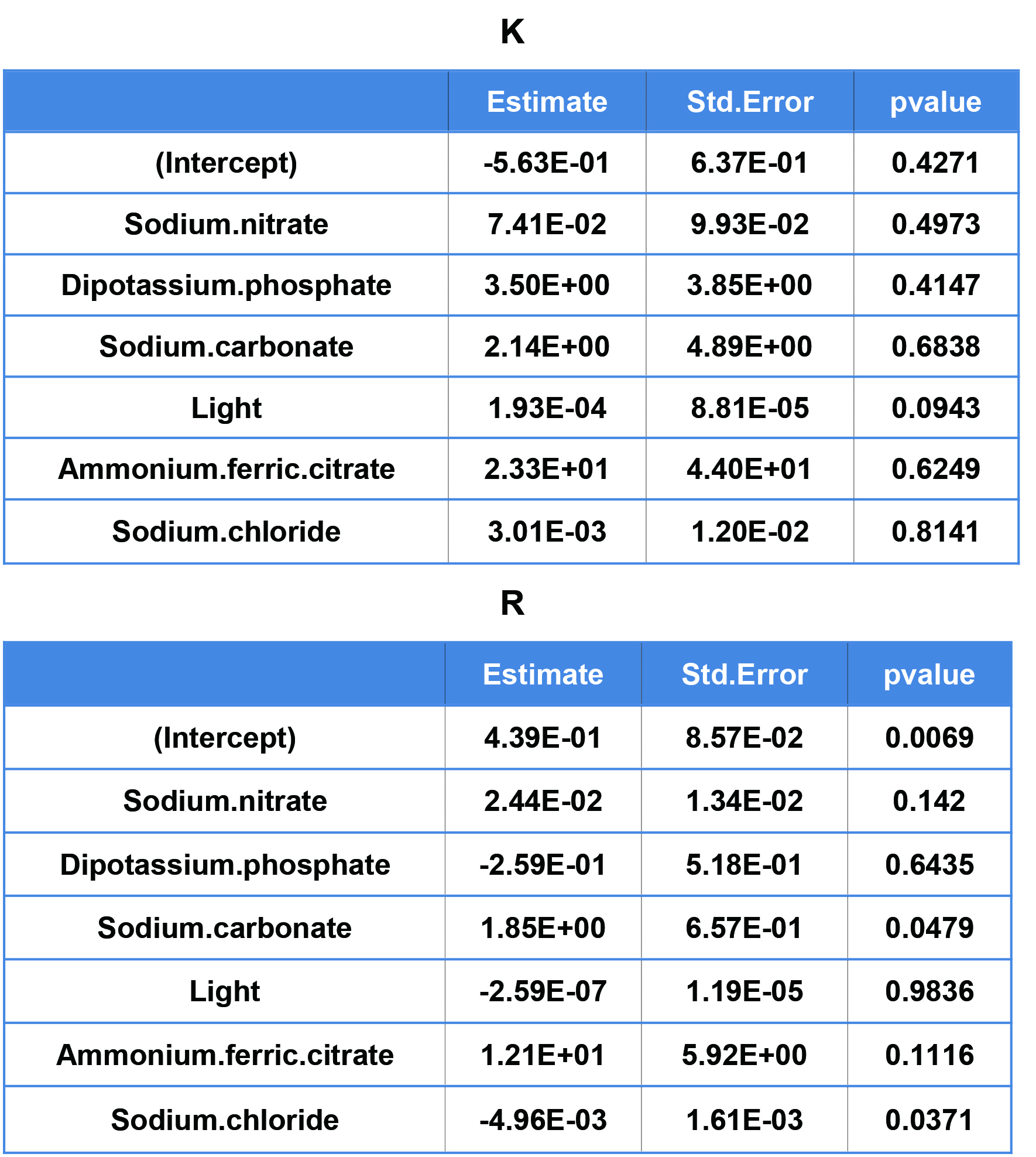We found that for parameter K, light is the only factor which present significant influence, and for parameter R, sodium carbonate, sodium chloride presented significant influence. The above information gives our basic understandings of culture condition influence to the growth of microbial cells.But we didn't find any significant variables influence the parameter N0. We used AIC to help us to select the best model to predict kinetic parameters. The Akaike information criterion(AIC) is an estimator of the relative quality of statistical models for a given set of data. Through AIC, we chose Sodium.nitrate and light to predict parameter K, and used Sodium.carbonate, Sodium.chloride, Ammonium.ferric.citrate, Sodium.nitrate to predict parameter R.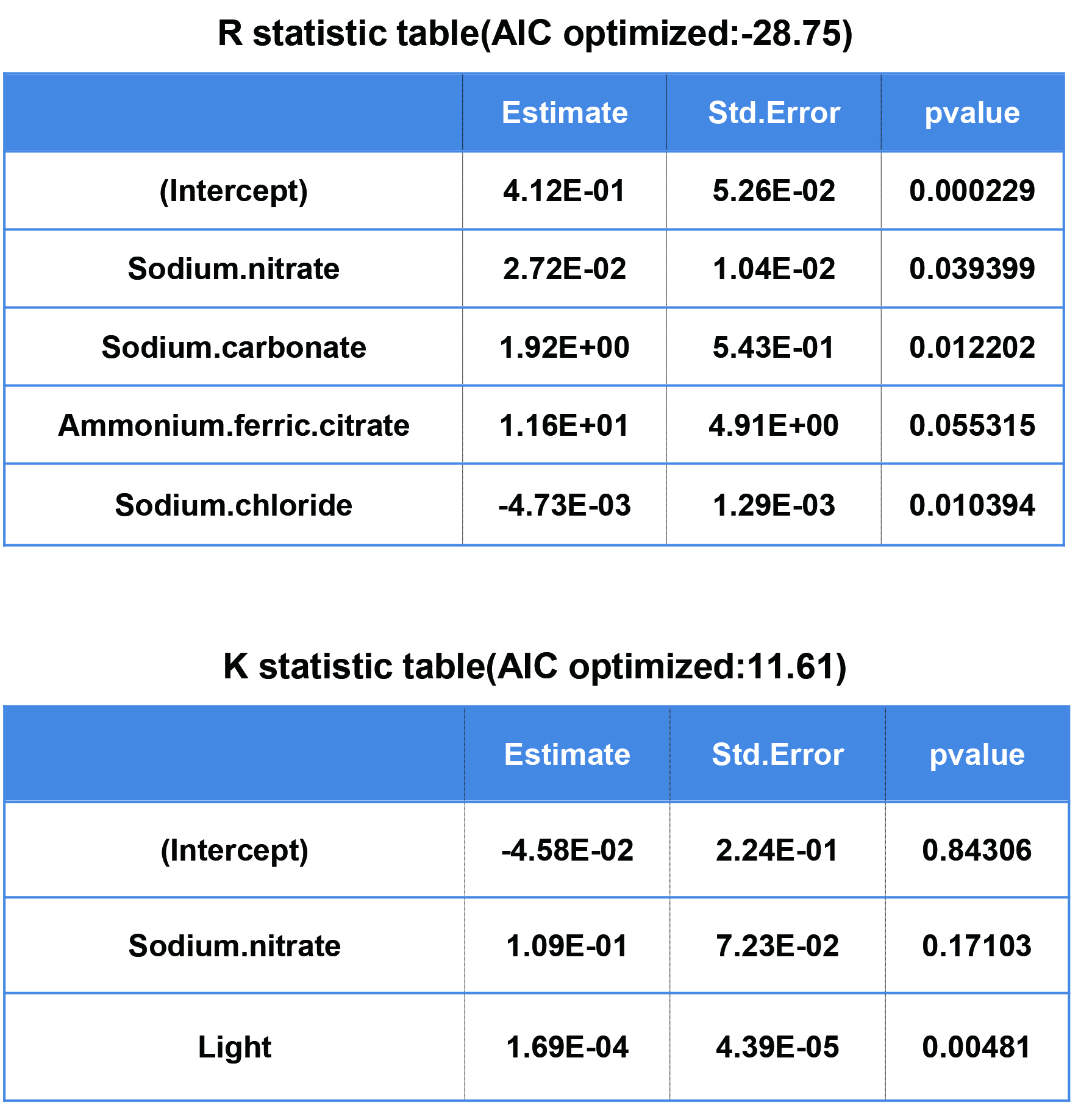Besides, our variables were not necessary need to linear correlates with parameters, We performed support vector machine, a well-known non-linear machine learning algorithm to improve the performance of prediction. We used leave-one-out cross validation(LOOCV) technique to compare the performance between linear regression model and SVM regression with the linear kernel.

Suppose we have n sample to training, every round we extracted one sample to predict and n-1 samples to train our model. Running above step for n times, we calculated R2 on test dataset defined as blow.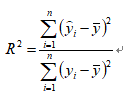Yi means the sample need to be predicted at the ith time. Through LOOCV, we found that SVM regression is perform better that linear regression model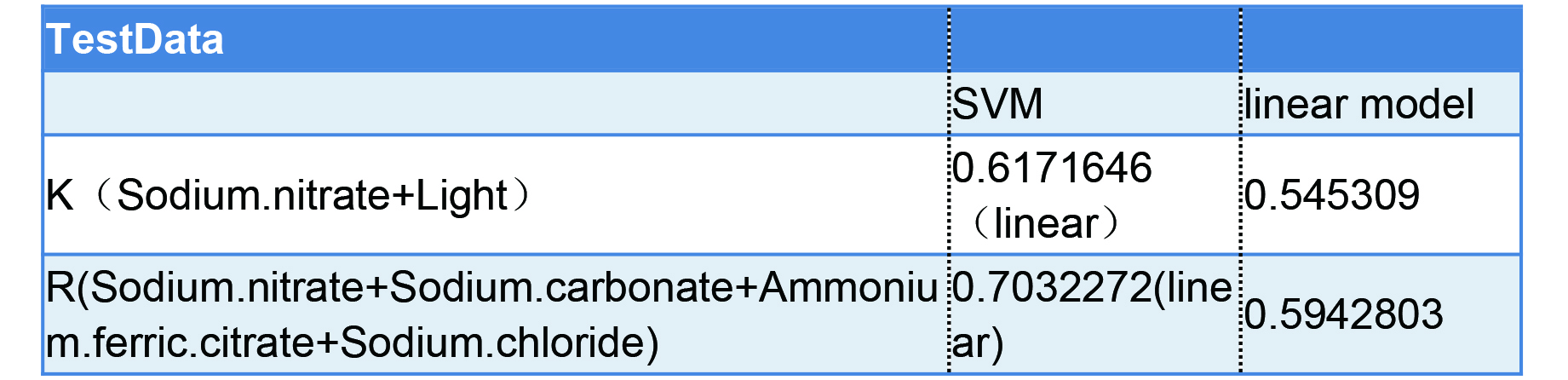## Sensitive analysis of parameter N0

In first layer training step we found that our culture condition variables can't predict parameter N0. To investigate the influence of parameter N0, we summarized the minimum and maximum N0 value of fitted 12 samples and combined with previous parameter K and R prediction model to predict the growth of all samples.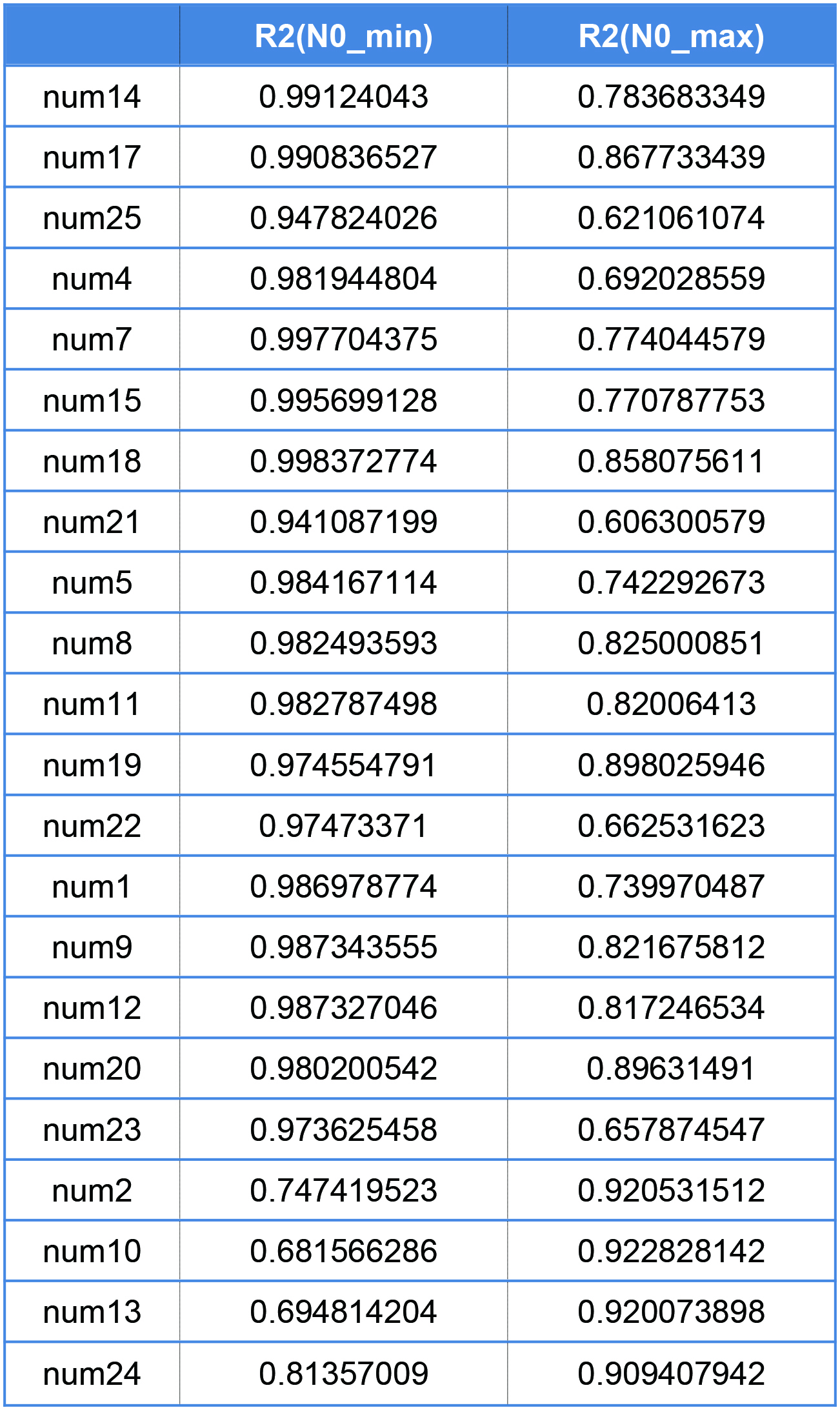We notice that using N0_min will not influence the fitness of samples with light condition < 9000 Lux, while in 9000 Lux group, using N0_max will present good fitness. Motived by it, when light =>9000 lux, we used N0_max to estimate N0, else used N0_min to estimate N0

## Simulation and prediction

We simulated about 1875 combinations which are not measured through our experiment and we found the following condition present the best K and R value compare with other schemes.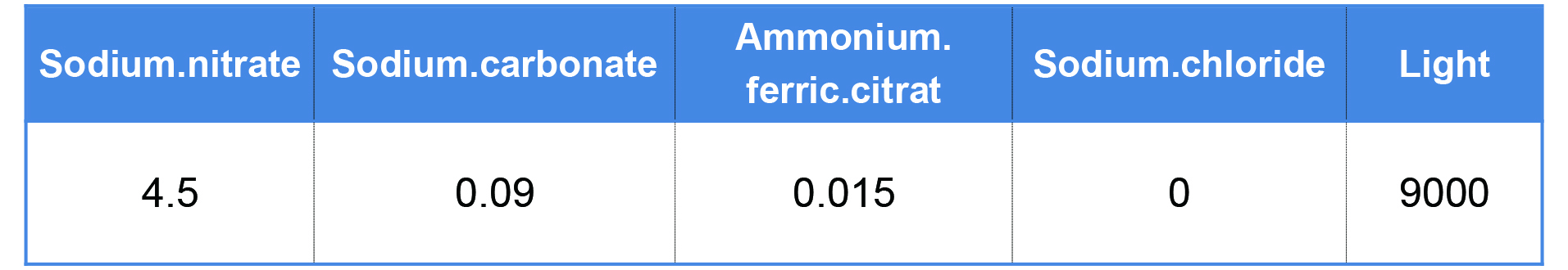Besides, we also simulated its growth curve and compared with our measured sample growth curve. Which showed that our culture conditions could be optimized.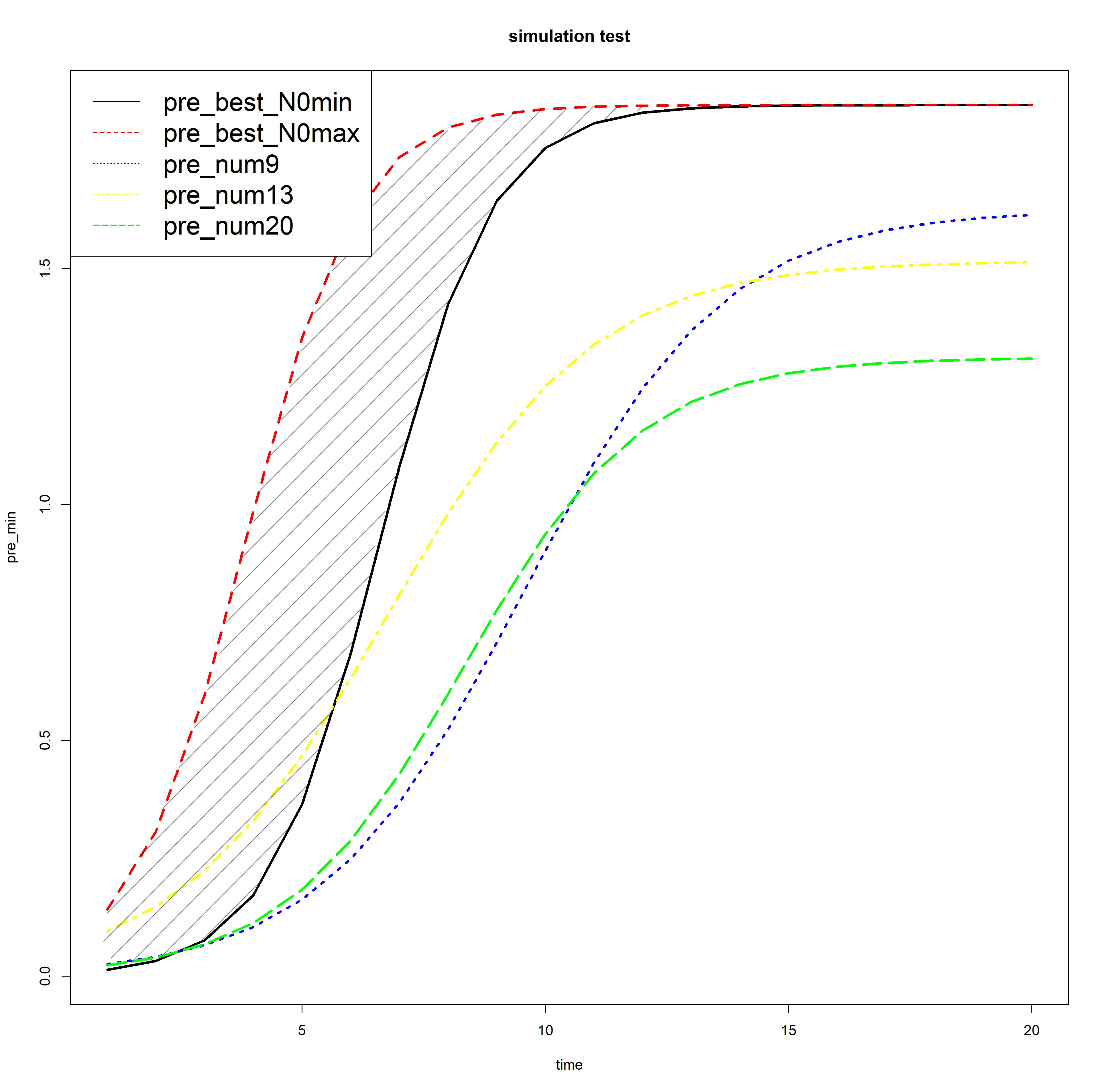Reference

 Karline Soetaert, Thomas Petzoldt, R. Woodrow Setzer (2010). Solving Differential Equations in R: Package deSolve. Journal of Statistical Software, 33(9), 1--25. URL http://www.jstatsoft.org/v33/i09/ DOI 10.18637/jss.v033.i09

School's name:SCAU

Member's name:SCAU

Designed by:SCAU